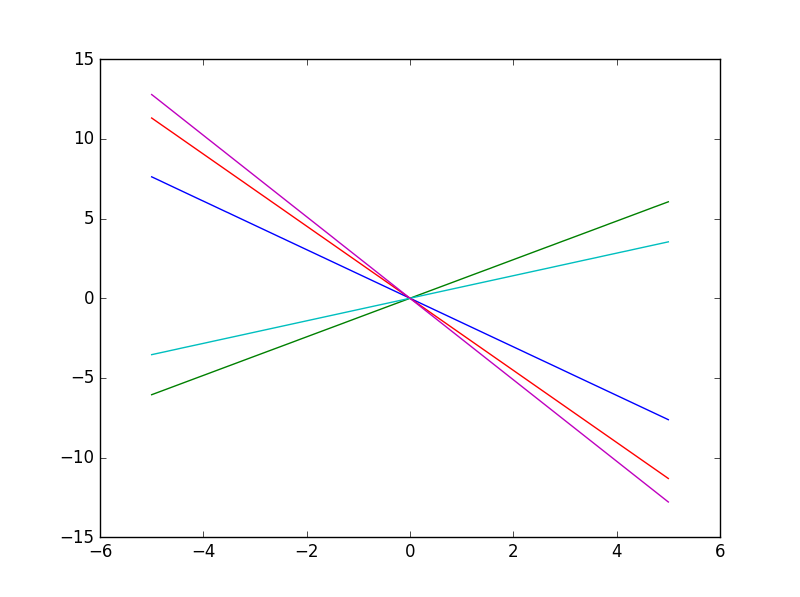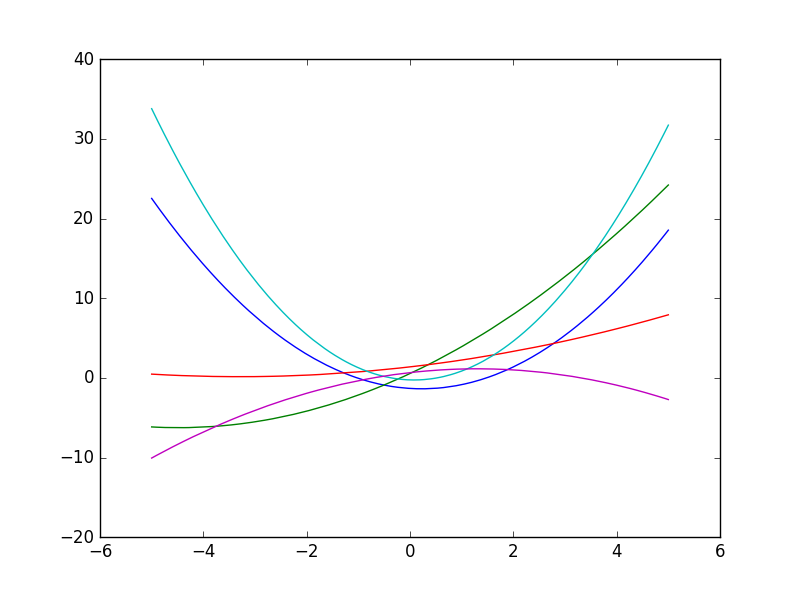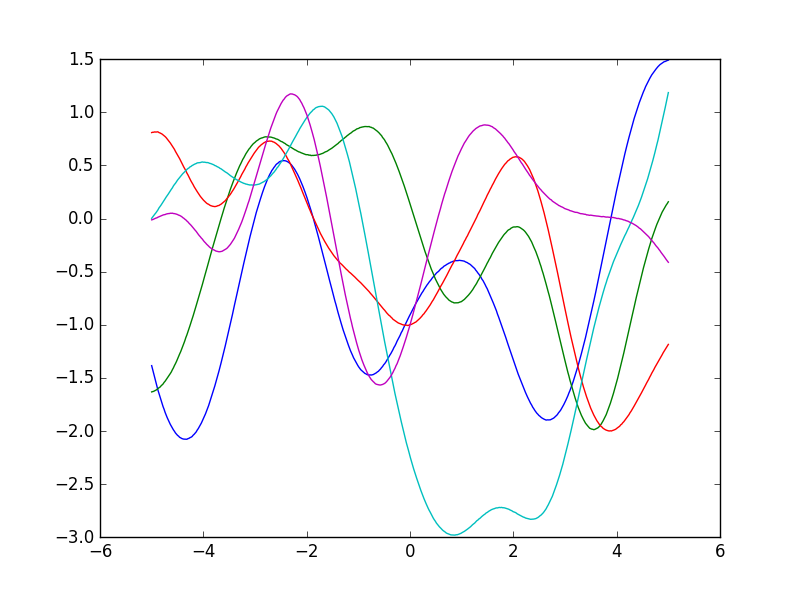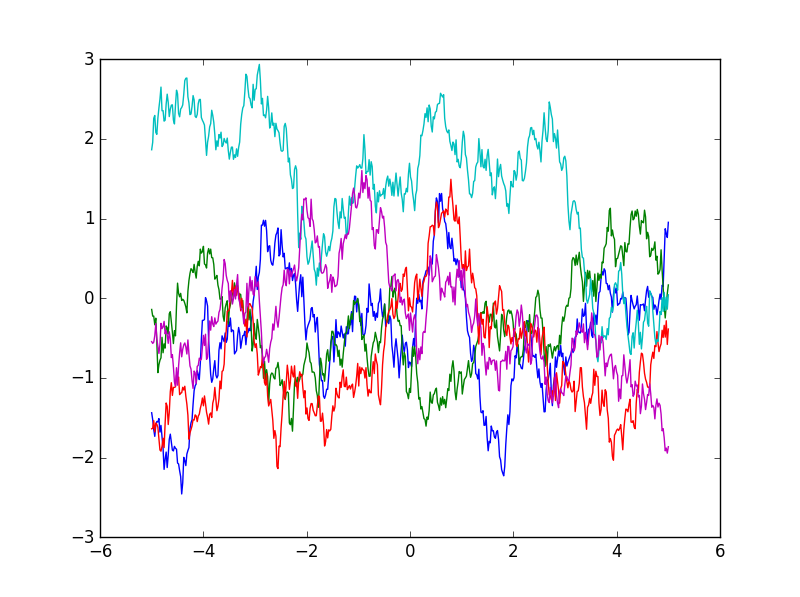# Gaussian processes¶

Gaussian processes model a probability distribution over functions.

Letbe some function mapping vectors to vectors. Then we can write:whererepresents the mean vector:andis the kernel function.

## Kernel function¶

The kernel is a function that represents the covariance function for the Gaussian process.The kernel can be thought of as a prior for the shape of the function, encoding our expectations for the amount of smoothness or non-linearity.

Not all conceivable kernels are valid. The kernel must produce covariance matrices that are positive-definite.

### Linear kernel¶Some functions sampled from a Gaussian process with a linear kernel:### Polynomial kernel¶Functions sampled from a Gaussian process with a polynomial kernel whereand:### Gaussian kernel¶

Also known as the radial basis function or RBF kernel.Some functions sampled from a GP with a Gaussian kernel:### Laplacian kernel¶Functions sampled from a GP with a Laplacian kernel:## Sampling from a Gaussian process¶

The method is as follows:

1. Decide on a vector of inputsfor which we want to compute, whereis some function which we will sample from the Gaussian process.
2. Compute the matrixwhere.
3. Perform Cholesky decomposition on, yielding a lower triangular matrix.
4. Sample a vector of numbers from a standard Gaussian distribution,.
5. Take the dot product ofand the vectorto get the samples.